#Function Repository Resource:

# PriorityQueue

Provide a priority queue data structure with its standard operations

Contributed by: Daniel Lichtblau
 ResourceFunction["PriorityQueue"][] returns a priority queue initialized with default settings. ResourceFunction["PriorityQueue"][{"InitialSize"→size,"NullElement"→null,"ComparisonFunction"→comp},"Initialize"] returns a priority queue initialized to have length size, null element null and comparison function comp. ResourceFunction["PriorityQueue"][inits,vals,"Initialize"] returns a priority queue initialized with parameters in inits and containing values vals. ResourceFunction["PriorityQueue"][queue,value,"Enqueue"] places value in queue. ResourceFunction["PriorityQueue"][queue,operation] performs the specified operation on queue.

## Details

Priority queues can be used for sorting by a given ordering function.
Priority queues are also used in the implementation of Prim's algorithm for computing minimum spanning trees.
The default values for "InitialSize", "NullElement" and "ComparisonFunction" are 16, Missing["empty slot"] and Greater, respectively.
"Initialize" can be omitted whenever the first argument is a list of three initialization settings.
In ResourceFunction["PriorityQueue"][queue,value,"Enqueue"], the explicit "Enqueue" can be omitted.
In ResourceFunction["PriorityQueue"][queue,operation], the allowed values of operation are "Length", "EmptyQ", "Dequeue" and "Peek", which perform the following actions:
 "Length" gives the number of elements currently in the queue "EmptyQ" gives True if the queue contains no elements, and gives False otherwise "Dequeue" removes the largest element from the queue and returns its value "Peek" gives the largest value without removing it from the queue
In ResourceFunction["PriorityQueue"][queue], the operation "Dequeue" is assumed. In typical usage, one would remove and store the largest element by doing topval=ResourceFunction["PriorityQueue"][queue,"Dequeue"]. Dequeuing all elements from a heap results in a list sorted according to the specified ordering function.
This implementation of priority queues uses binary heaps; this is a common technique.
In binary heap implementations such as this one, each element in a priority queue has either zero, one or two children. If the jthelement has children, they will be located at positions 2j and 2j+1 (with the latter position empty if there is ony one child). Elements are contiguous in the sense that there is never a hole (empty slot) until after the last element.
Each child is ordered less than its parent, but the children do not satisfy a prescribed order with respect to one another.
A priority queue is similar to a binary tree with breadth-first ordering (children considered lower than parents), except children are not always placed in their relative ordering.
Generally, a priority queue is an array of fixed size, so adding and removing elements do not incur memory allocation or deallocation.
The one exception is when adding an element to a queue that is at capacity, at which point the heap size is doubled.
ResourceFunction["PriorityQueue"] has the attribute HoldFirst.

## Examples

### Basic Examples (9)

Initialize a priority queue (aka ordered heap) with 20 random reals between -1 and 1:

 In:=Check the size:

 In:=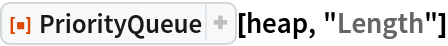Out=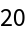Check the value of the largest element:

 In:=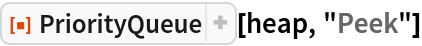Out=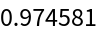Add 80 more random values to the heap:

 In:=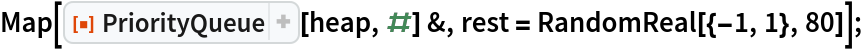Recheck the length and largest value:

 In:=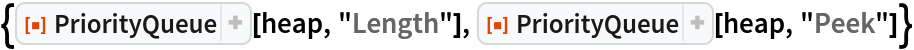Out=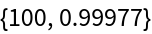When we dequeue elements one-by-one, the result is a sorted list of values; this method is called the "heap-sort" algorithm:

 In:=Out=Check that it is indeed empty:

 In:=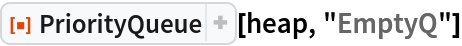Out=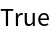An alternative check is that the length is zero:

 In:=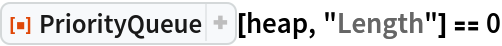Out=Check that the result is correctly sorted largest-to-smallest:

 In:=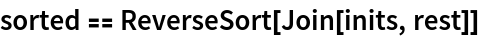Out=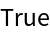### Scope (2)

PriorityQueue can work with any data type for which an ordering exists, such as words with alphabetical order:

 In:=Now sort them in dictionary order by dequeuing:

 In:=Out=### Properties and Relations (5)

For a queue of size n, we expect speed proportional to nlog(n) for creating and removing all elements. We test this by timing a basic sorting, then doubling the size twice.

Use a queue to enqueue 10,000 random values:

 In:=Out=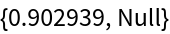Now dequeue all elements:

 In:=Out=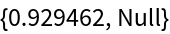Check that these are actually sorted from largest to smallest:

 In:=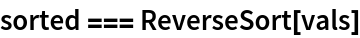Out=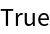Double the size and check enqueing and dequeing timings:

 In:=Out=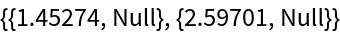Again double the size and check enqueing and dequeing timings:

 In:=Out=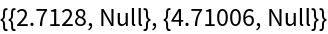### Possible Issues (1)

Attempting to dequeue from an empty queue gives the default element:

 In:=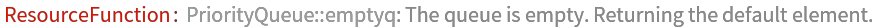Out=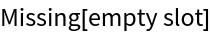### Applications (2)

#### Minimum Spanning Trees: Prim's Method (lazy version) (2)

A minimum spanning tree for a connected graph is a tree that connects every vertex, has no cycles and is of minimal total edge weight. The "lazy" version of Prim's method for computing the minimum spanning tree of a connected, weighted undirected graph works as follows. Start with the most minimal edge. Add its neighbors to a priority queue. Iteratively remove the shortest edge in the queue. If its second vertex is not yet in the tree under construction then add it to the tree and add all its edges to vertices not yet in the tree to the queue. This method was discovered independently by Jarník and Dijkstra. It has algorithmic complexity O(e log e), where e is the number of edges. Here we apply Prim's method for the special case of a complete graph given by a set of points in the plane, with edge weights being the distances between vertices.

Code for Prim's algorithm:

 In:=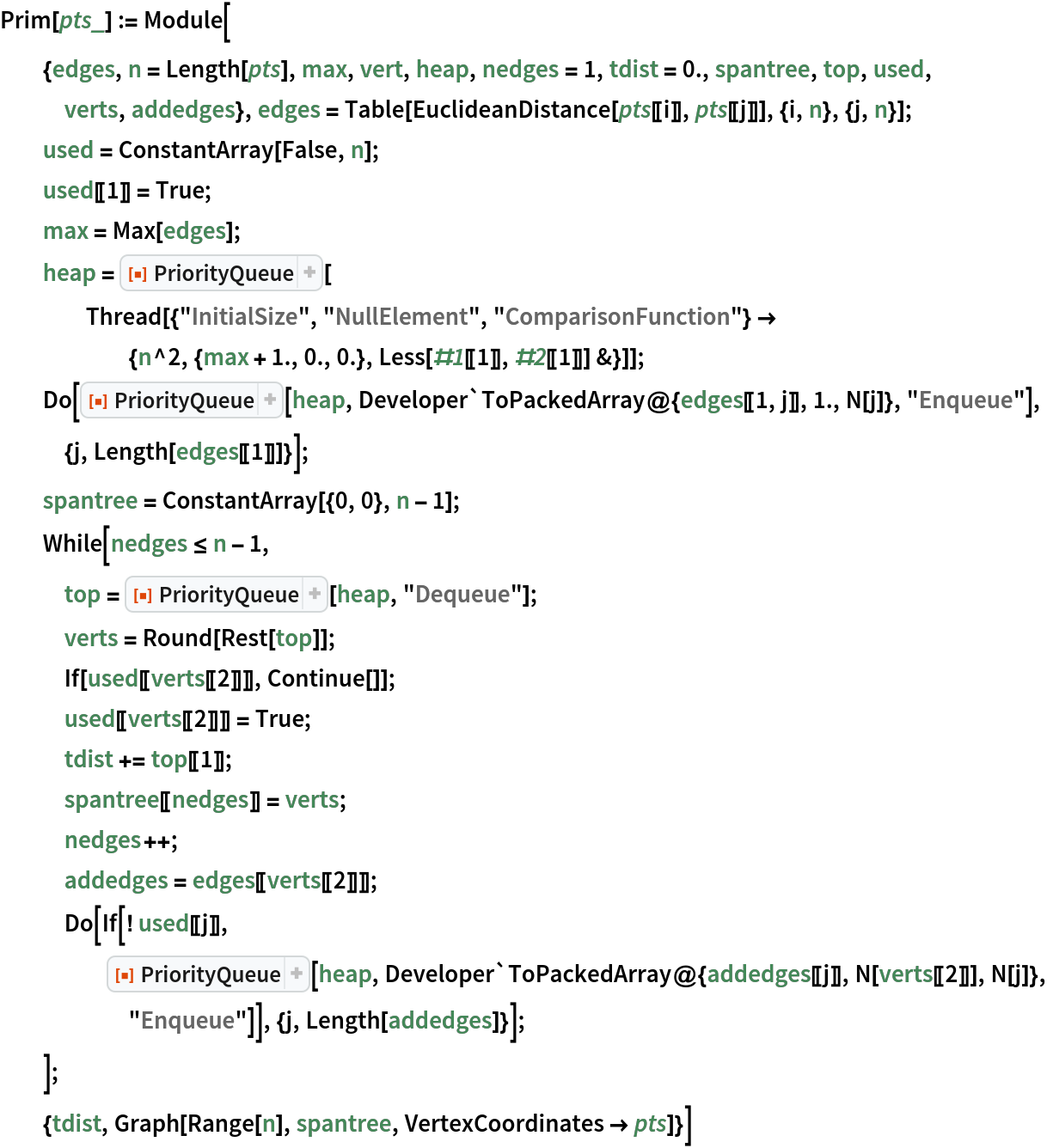Create random points in the plane:

 In:=Out=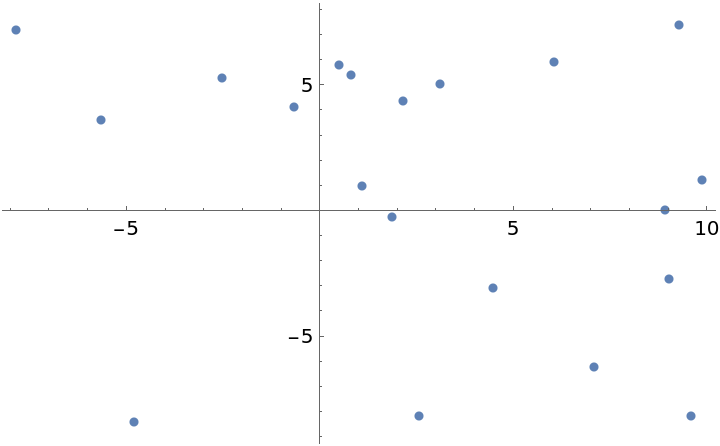Use Prim's method to compute their minimum spanning tree:

 In:=Out=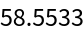Show the graph of the spanning tree:

 In:=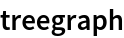Out=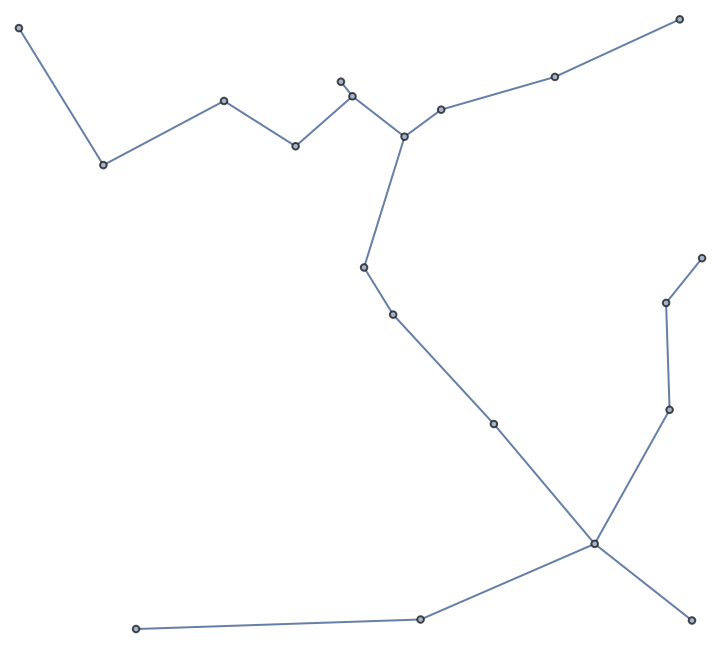For a graph with e edges, the time complexity of the lazy version using a priority queue is O(e log e). The worst-case space requirement is O(e). In these examples, we work with complete graphs, so with n vertices one has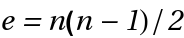edges.

Use Prim's method on a larger point set:

 In:=Out=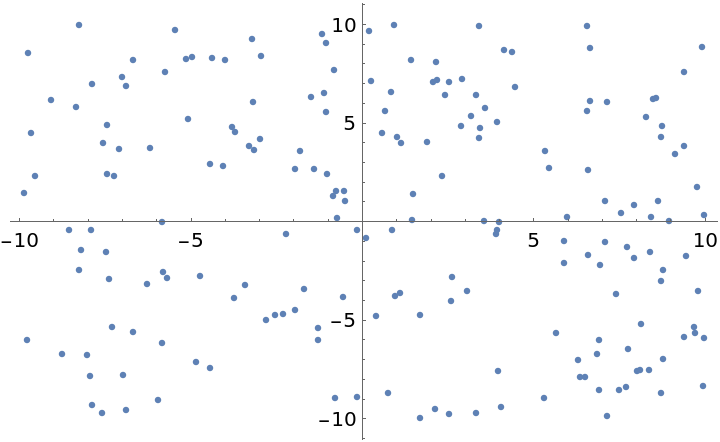Compute the minimal spanning tree:

 In:=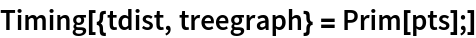Out=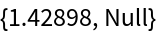Show the total branch length of this spanning tree:

 In:=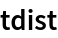Out=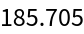Show the spanning tree:

 In:=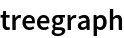Out=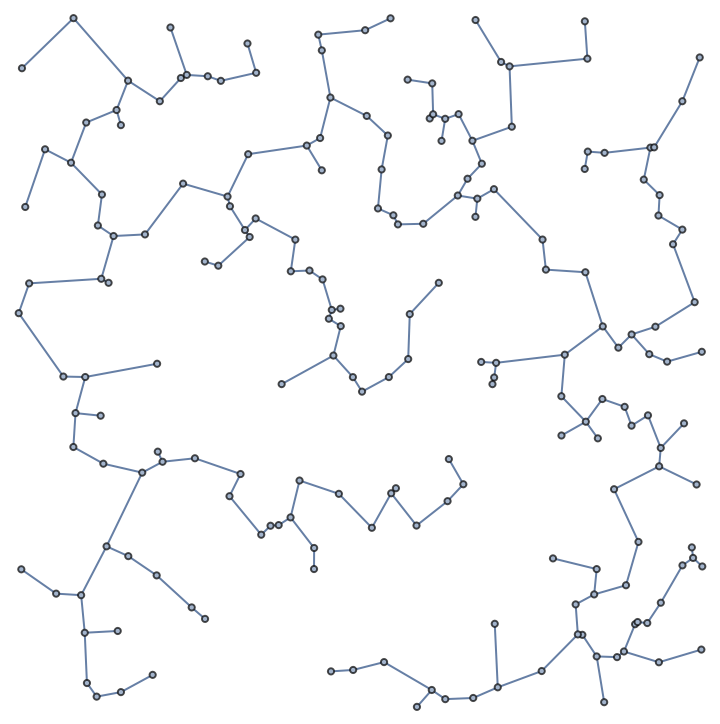Double the size of the point set:

 In:=Out=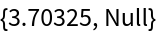Show the spanning tree:

 In:=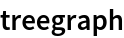Out=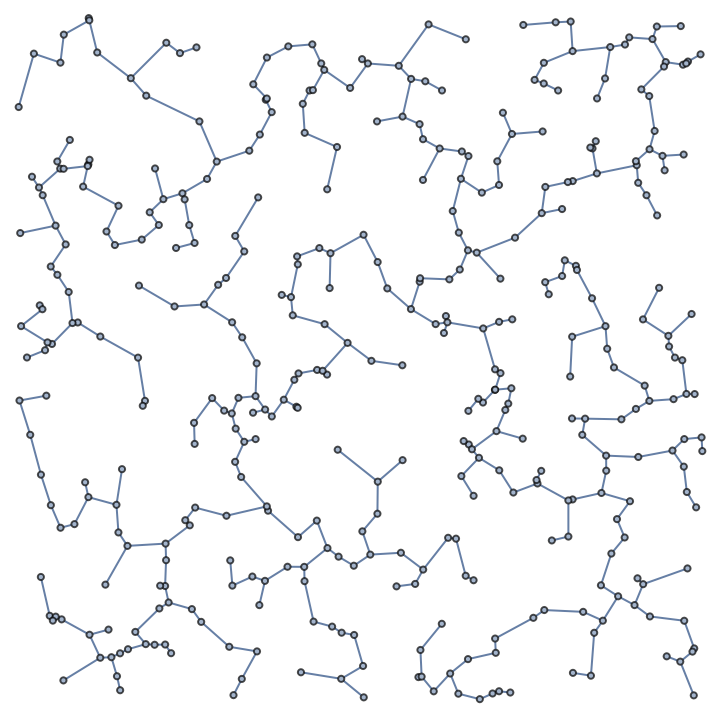The Prim's method built into FindSpanningTree has the same asymptotic behavior but is many times faster:

 In:=Out=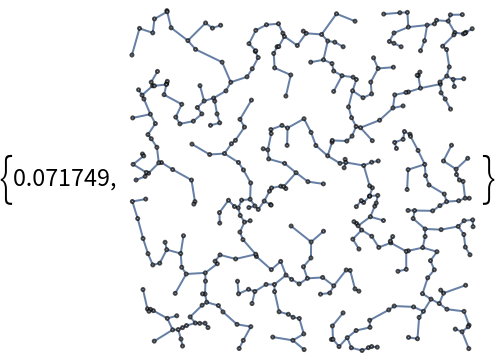## Author Notes

A built-in version of this is available as DataStructure["PriorityQueue"]